# Basic Visualization for Civic Tech

A major part of civic tech is the ability to visualize data. The ability to create maps, graphs, and charts quickly and effectively improves the ability of a civic tech community to help tell the story of their community through data.

One excellent way to create visualizations is through the ggplot2 package. Using R and data provided through the Delaware Open Data Portal, we can build an example of a simple visualization that shows the power of ggplot2.

First, let’s take a look at the Public Library Usage dataset. For now, we’ll just download the .csv. In future iterations, we could directly connect to the open data portal API provided by Socrata.

## Data Exploration Techniques

After we import the data into R, we can begin exploring the data. There are a few simple commands that are useful for exploring data in R. Besides these, there are many more that are useful, but you can start to get a feel for the data by using these methods.

```# See a summary of the structure of the data
str(Public_Library_Usage)

# See a summary of the data
summary(Public_Library_Usage)

# The number of rows
nrow(Public_Library_Usage)

# The number of columns
ncol(Public_Library_Usage)

# Column names
colnames(Public_Library_Usage)

# See the first six rows of data

# See all of the data
View(Public_Library_Usage)```

## Exploring Individual Features and Observations

When we’re exploring the data, we might also want to look at individual features (columns) and observations (rows) in the dataset. There are a few different ways to do this in R.

```# See an individual column by calling it by name
Public_Library_Usage\$`Fiscal Year`

# Try another one
Public_Library_Usage\$`Total Visits`

# See an individual column by using brackets
Public_Library_Usage[,1]

# See multiple columns in a row by using a colon
Public_Library_Usage[,1:3]

# See selected columns by using c()
Public_Library_Usage[,c(1,3,5,7)]

# See rows by using brackets
Public_Library_Usage[1,]

# All of the same things that we did to columns apply to rows
Public_Library_Usage[1:50,]
Public_Library_Usage[c(4,8,15,16,23,42),]

# Combinations of those things work as well
Public_Library_Usage[1:50,1:5]

# We can store the data into another "object" using "<-"
Public_Library_Usage_mini <- Public_Library_Usage[1:50,1:5]

# We can now use this as shorthand in our code
View(Public_Library_Usage_mini)```

## Graphing Data

While it’s always helpful to explore data by looking at it, much more information is gained by visualizing it with graphs. The base package of R has decent tools for quick visualizations, but if a more complex and polished graph is needed, then ggplot2 is the tool that is really needed.

Let’s use the Public Library Usage dataset to explore the relationship between Total Visits to the library, Juvenile Circulation, the Fiscal Year, and Computer Usage.

Before we actually begin, here’s an overview of what we’ll be doing in each step. Details and individual explanations of those steps follow below.First, make sure that ggplot2 is set to be used. If you’re unfamiliar with R, this can be accomplished with 2 simple commands.

```# First, we install the package. This only needs to be done once on a given computer
install.packages("ggplot2")

# Next we tell R to use the package
require(ggplot2)```

ggplot2 is based on the idea of “Grammar of Graphics”.  Elements are layered on top of each other in order to create a cohesive plot. There are three key components of any plot: the data, “geoms”, and a coordinate system. The data is mapped onto different “aesthetic” properties. We’ll use several of these in order to create our plot of the Public Library Usage dataset.

Before we start our exploration, we’ll need to make one small change to our dataset. You might have noticed in your exploration that many of the column names had spaces. Unfortunately, ggplot2 cannot handle this. Thankfully, there’s a very simple command that we can use to fix the problem.

`colnames(Public_Library_Usage) <- gsub(" ","_",colnames(Public_Library_Usage),fixed=TRUE)`

This replaces all of the column names in the dataset so that every space is replaced with an underscore. This bypasses the issue that we had and allows us to move forward with our plotting.

Here’s the basic framework of a ggplot2 visualization.

`Public_Library_base_graph <- ggplot(data=Public_Library_Usage,aes(x=Total_Visits,y=Juvenile_Circulation))`

The statement is split up into several pieces. First, we declare the dataset that we are using. In our case, that is “Public_Library_Usage”. Next, we declare the aesthetics that the data should be mapped to. This is done using the “aes()” parameter. We pass two features into this parameter. One determines the x-axis, “Total_Visits”, and the other determines the y-axis, “Juvenile_Circulation”. Last, this is all stored into another object that we call “Public_Library_base_graph”.

This does not create a graph in itself, but rather lays down the base that a graph can be built on top of. A geom needs to be built on top of the base in order to get a graph. You can find a full cheat sheet of geoms here.

```Public_Library_base_graph +
geom_point()```

Because we stored the ggplot object into Public_Library_base_graph, we are able to use it again. Everything else can be built off of this object without having to restate everything else. Each new layer of the visual is added in using a “+” sign. The geom allows for us to build our first graph.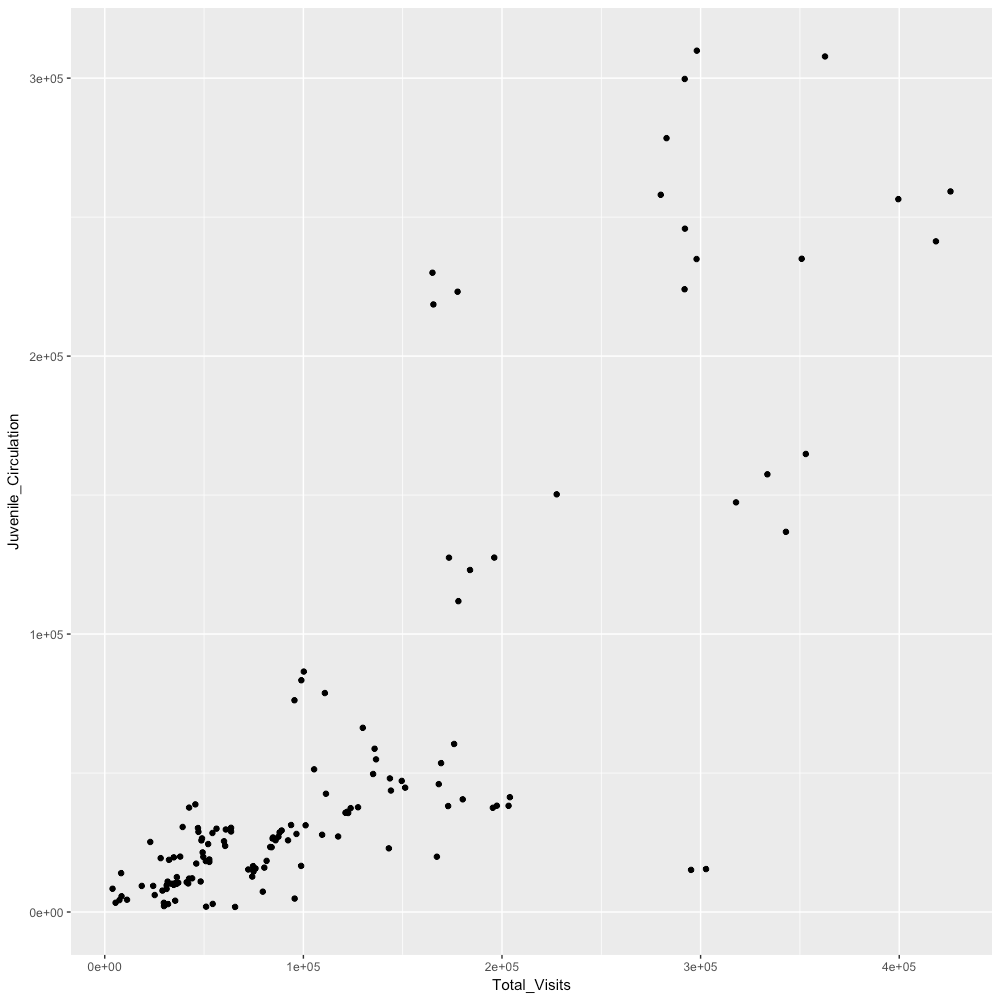This is not a particularly enticing graph yet and only covers 2 of the 4 features that we intend to represent. Let’s add in the next features.

```Public_Library_base_graph +
geom_point(aes(colour=Fiscal_Year))```

Passing another aes() parameter allows us to use “Fiscal_Year” as another feature. Specifically, the Fiscal_Year will dictate the change in color based upon the year of the data point.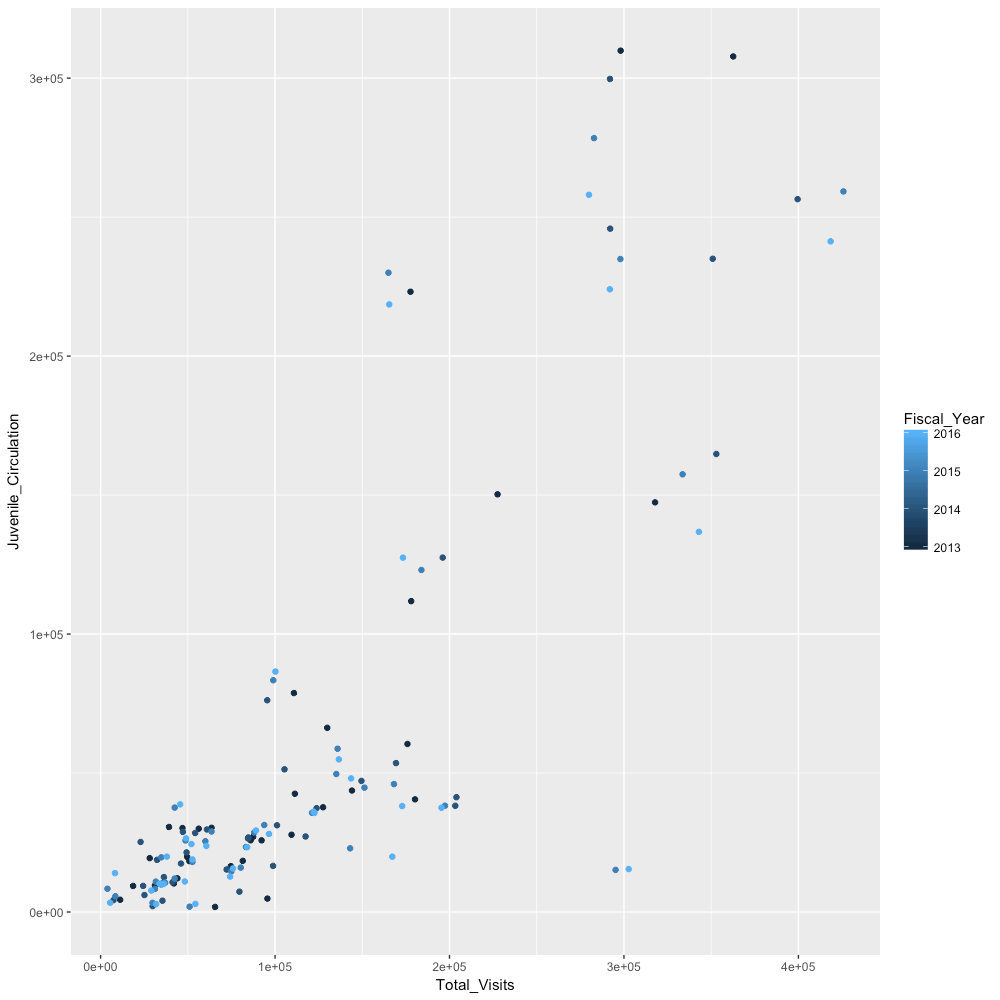Doing this automatically adds in a legend based upon each year that is available. Notice that it is currently being represented as a scale. The year is not a scale, though. Rather, it is a factor. Our next iteration will allow us to change this.

```Public_Library_base_graph +
geom_point(aes(colour=factor(Fiscal_Year)))```

The addition of “factor” corrects this problem.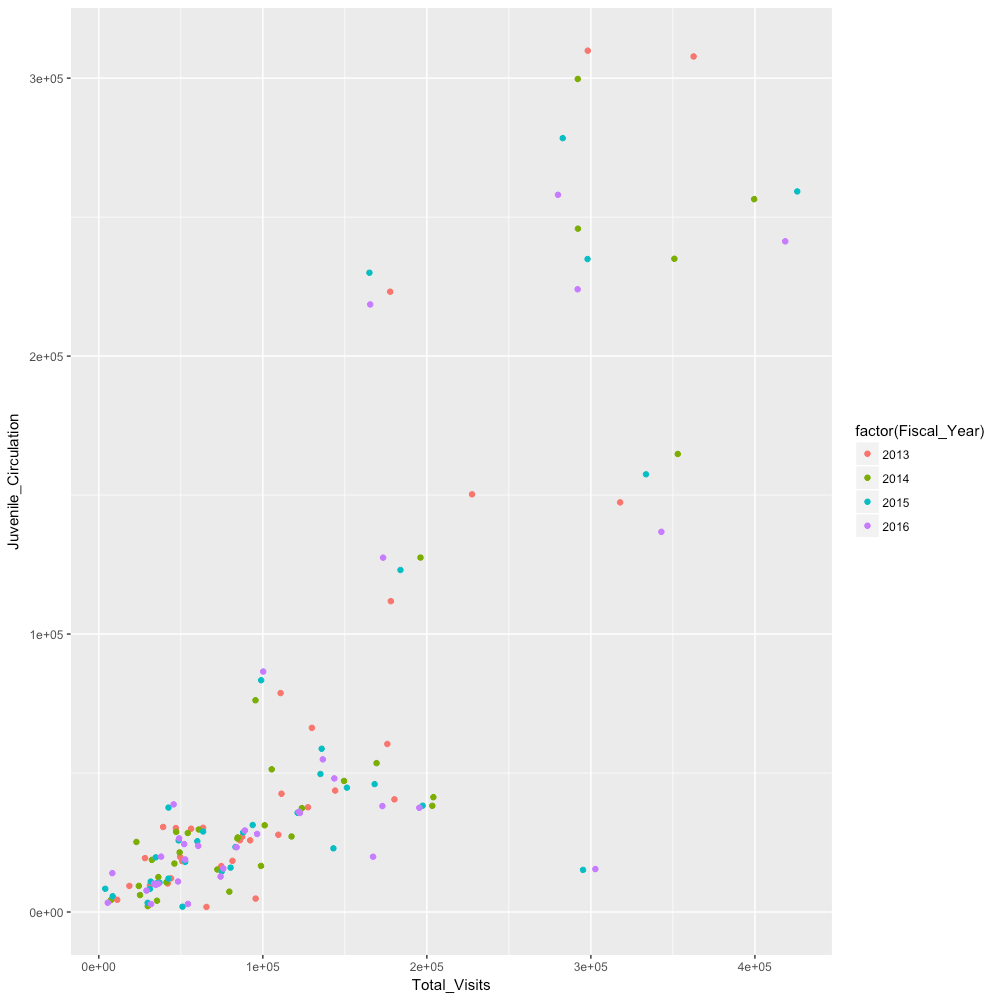It’s certainly much easier to see the difference between years now. However, we can improve the graph by adding in a bit of transparency to the points. This would make it easier to see some of the points that are clustered together. We can accomplish this by passing one more parameter into geom_point.

```Public_Library_base_graph +
geom_point(aes(colour=factor(Fiscal_Year),
size=Computer_Usage),
alpha=0.5)```

The alpha parameter accepts a value between 0 and 1. A value of 0 would be entirely transparent, whereas a value of 1 is entirely opaque. The 0.5 that we use makes it easier to see the clumped up data points.Now, let’s take care of the other feature that we want to add, Computer_Usage. We’ll pass one more value into the aes() parameter of geom_point. Specifically, we’ll tie its value to the size of the point.

```Public_Library_base_graph +
geom_point(aes(colour=factor(Fiscal_Year),
size=Computer_Usage),
alpha=0.5)```Now we have all of the features that we want mapped to the data and we can begin making the graph look more professional. We can start by adding in features that we’re used to seeing on graphs…like labels. This is easy to do by adding in another layer.

```Public_Library_base_graph +
geom_point(aes(colour=factor(Fiscal_Year),
size=Computer_Usage),
alpha=0.5) +
ggtitle("Total Visits vs Juvenile Circulation")```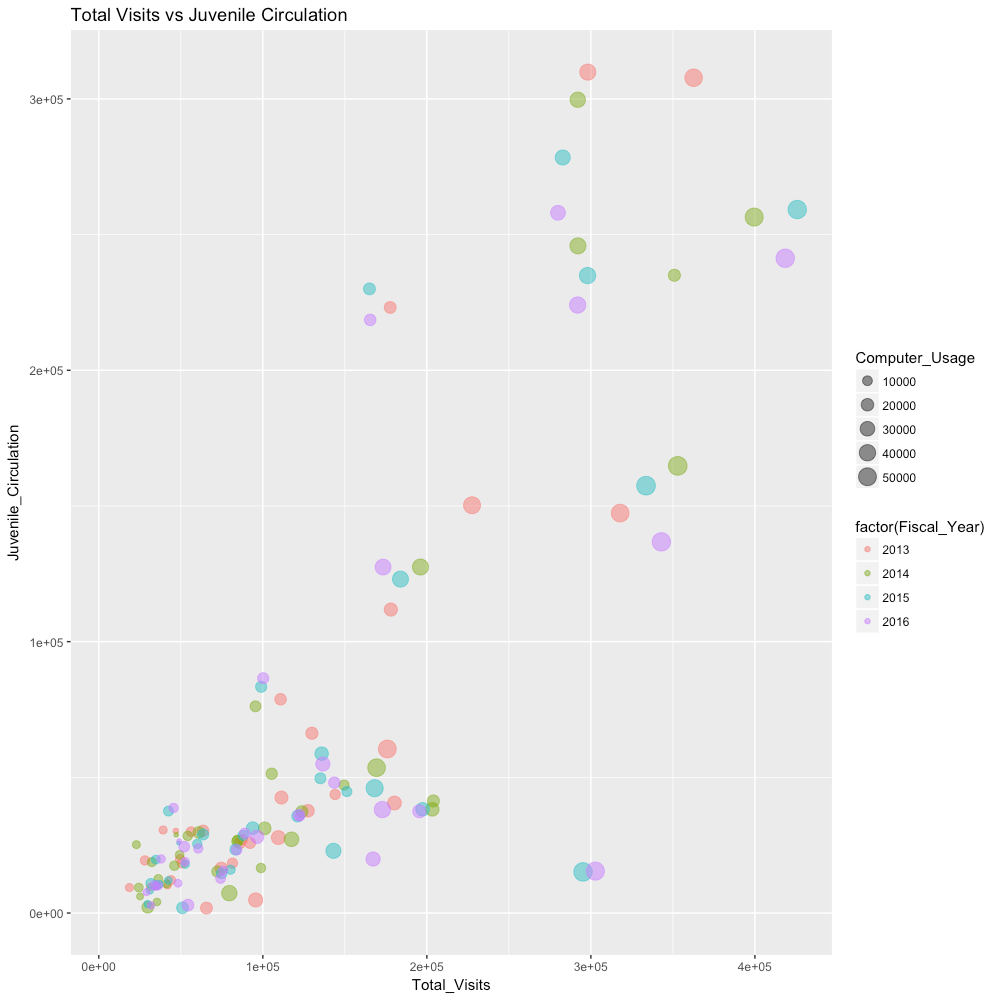The use of ggtitle allows us to put in both a title and a subtitle. The first parameter creates the title and the second creates the subtitle. We’ll add a subtitle in that describes what happens as the size of the bubble increases.

```Public_Library_base_graph +
geom_point(aes(colour=factor(Fiscal_Year),
size=Computer_Usage),
alpha=0.5) +
ggtitle("Total Visits vs Juvenile Circulation",
"Size of bubble is dictated by amount of Computer Usage")```Now, there are a few different things that we should take care of. First, while we needed the underscores in order to make it possible to graph, they look poor for a final graph. While we’re cleaning the axis labels up, we should also look at how to change the tick mark labels. Also, the legend for Fiscal_Year needs to be relabeled. It doesn’t look particularly becoming at the moment. Last, the subtitle already states what’s happening with bubble size. We no longer need that particular legend, so it should be eliminated.

First, the labels are added in by layering on xlab and ylab.

```Public_Library_base_graph +
geom_point(aes(colour=factor(Fiscal_Year),
size=Computer_Usage),
alpha=0.5) +
ggtitle("Total Visits vs Juvenile Circulation",
"Size of bubble is dictated by amount of Computer Usage") +
xlab("Total Visits") +
ylab("Juvenile Circulation")```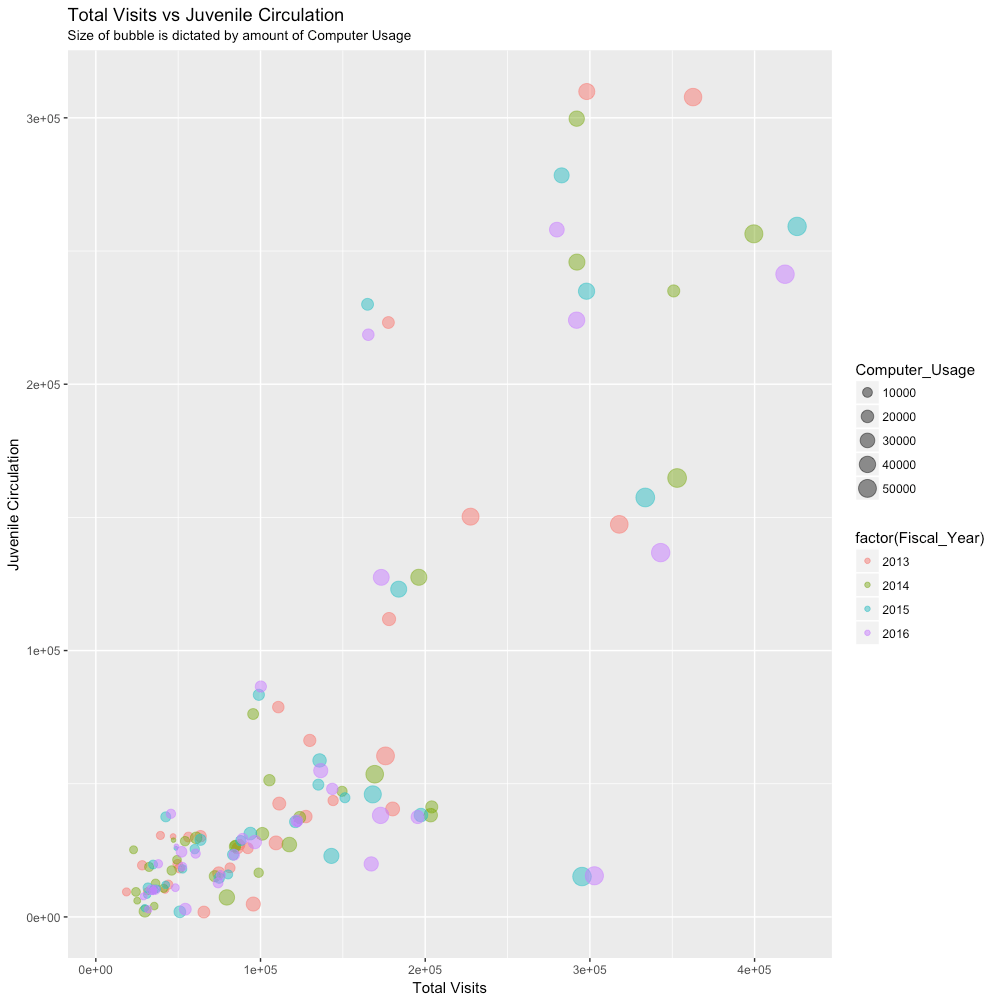Next, the tick marks are adjusted using scale_x_continuous and scale_y_continuous.

```Public_Library_base_graph +
geom_point(aes(colour=factor(Fiscal_Year),
size=Computer_Usage),
alpha=0.5) +
ggtitle("Total Visits vs Juvenile Circulation",
"Size of bubble is dictated by amount of Computer Usage") +
xlab("Total Visits") +
ylab("Juvenile Circulation") +
scale_x_continuous(labels = scales::comma) +
scale_y_continuous(labels = scales::comma)```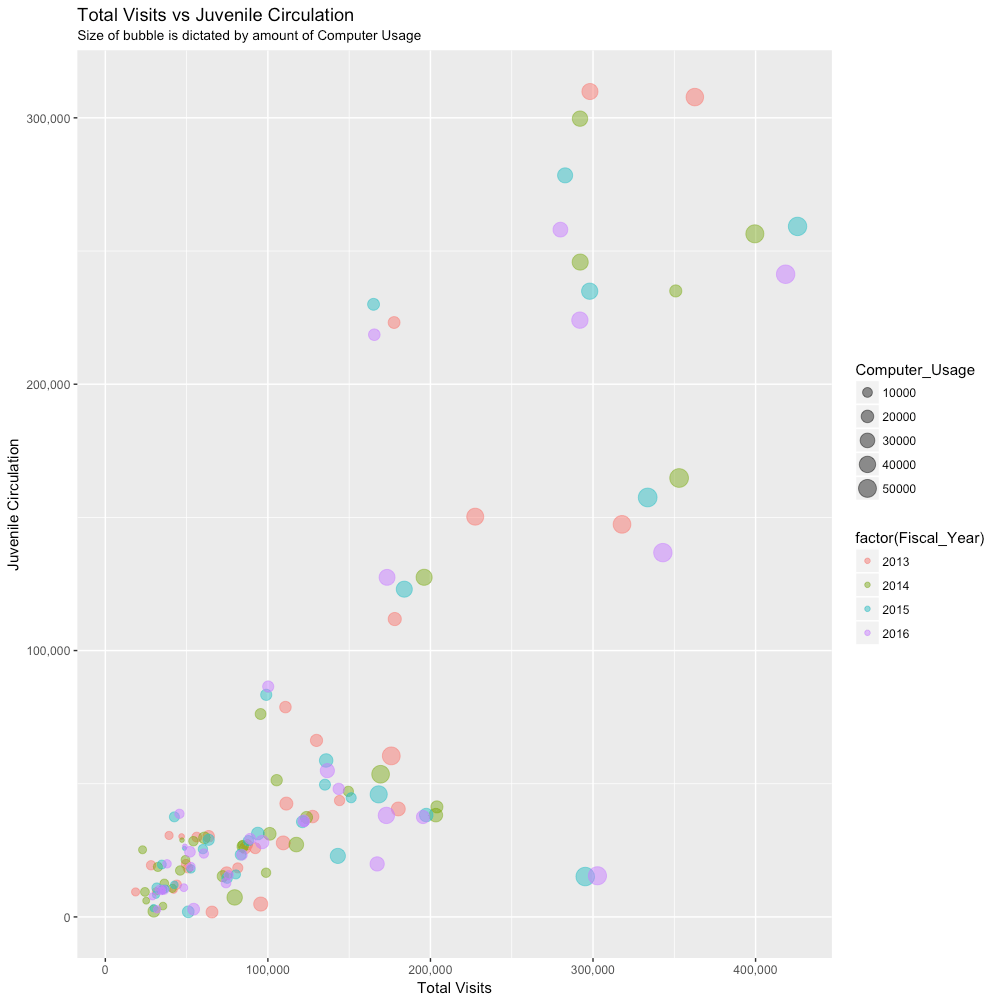Now we remove the legend for Computer_Usage by using “scale_size”. Each legend has a similar name. They can be removed (or adjusted) by referencing them.

```Public_Library_base_graph +
geom_point(aes(colour=factor(Fiscal_Year),
size=Computer_Usage),
alpha=0.5) +
ggtitle("Total Visits vs Juvenile Circulation",
"Size of bubble is dictated by amount of Computer Usage") +
xlab("Total Visits") +
ylab("Juvenile Circulation") +
scale_x_continuous(labels = scales::comma) +
scale_y_continuous(labels = scales::comma) +
scale_size(guide="none")```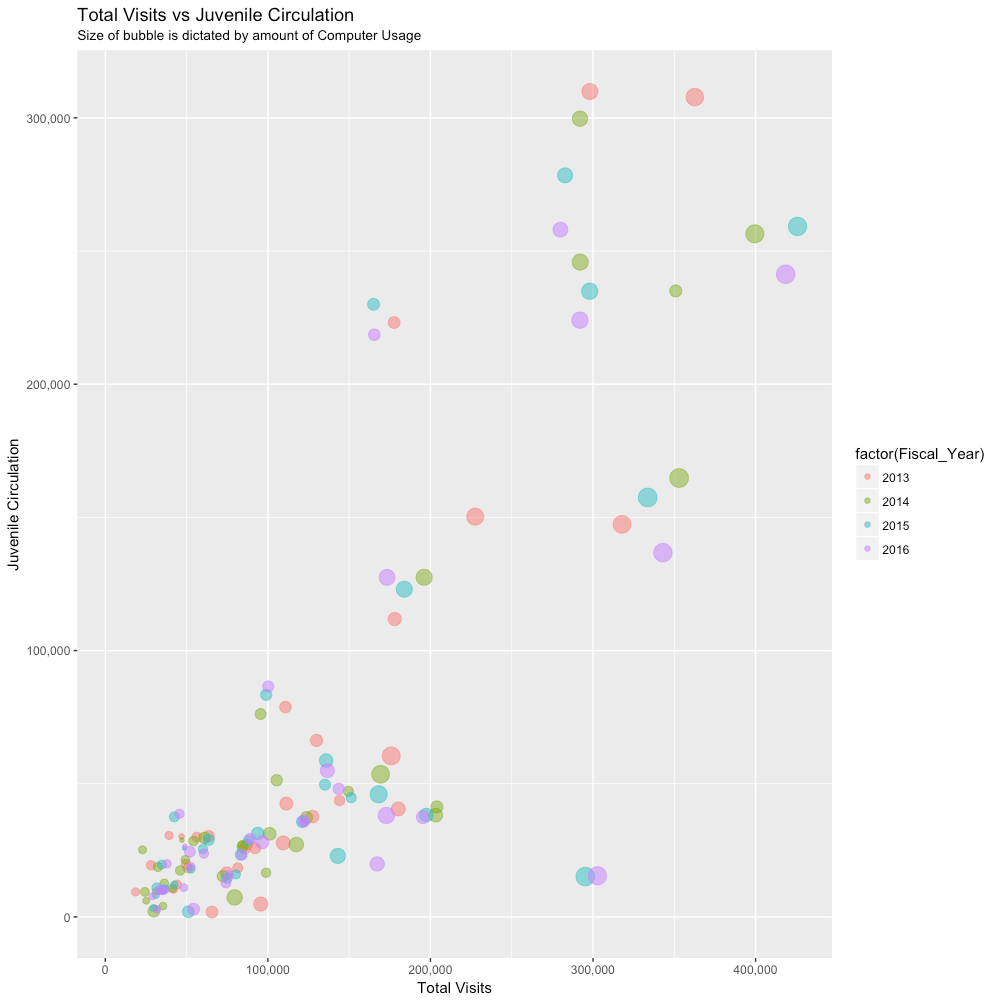Finally, we relabel “factor(Fiscal_Year)” using a similar method to what we used to remove the “Computer_Usage” legend.

```Public_Library_base_graph +
geom_point(aes(colour=factor(Fiscal_Year),
size=Computer_Usage),
alpha=0.5) +
ggtitle("Total Visits vs Juvenile Circulation",
"Size of bubble is dictated by amount of Computer Usage") +
xlab("Total Visits") +
ylab("Juvenile Circulation") +
scale_x_continuous(labels = scales::comma) +
scale_y_continuous(labels = scales::comma) +
scale_size(guide="none") +
scale_colour_discrete(name="Fiscal Year")```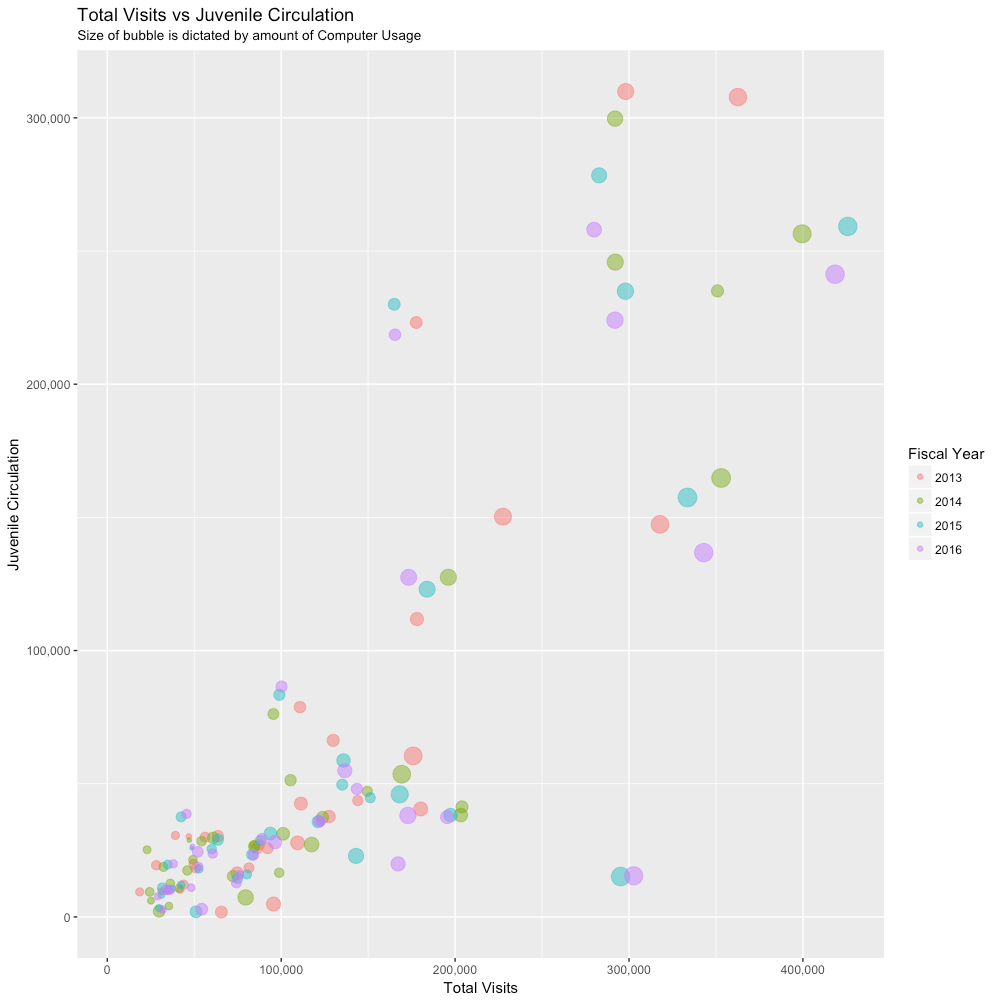Now, this is much better than where we were before, but it still isn’t particularly attractive. Thankfully, ggplot2 has a themes function. Nearly every bit of the plot can be superficially changed using themes. We’ll do all of these in one pass.

```Public_Library_base_graph +
geom_point(aes(colour=factor(Fiscal_Year),
size=Computer_Usage),
alpha=0.5) +
ggtitle("Total Visits vs Juvenile Circulation",
"Size of bubble is dictated by amount of Computer Usage") +
xlab("Total Visits") +
ylab("Juvenile Circulation") +
scale_x_continuous(labels = scales::comma) +
scale_y_continuous(labels = scales::comma) +
scale_size(guide="none") +
scale_colour_discrete(name="Fiscal Year") +
theme(
plot.title = element_text(family = "Roboto", color="#000000", face="bold", size=14, hjust=0),
plot.subtitle = element_text(family = "Roboto", color="#4c4d4f", size=9, hjust=0),
axis.title = element_text(family = "Roboto", color="#4c4d4f", face="bold", size=12),
panel.grid.major.x = element_blank(),
panel.grid.major.y = element_blank(),
panel.grid.minor.y = element_blank(),
panel.background = element_blank(),
axis.line.x = element_line(color = "black"),
axis.line.y = element_line(color = "black"),
aspect.ratio = 1/1
)```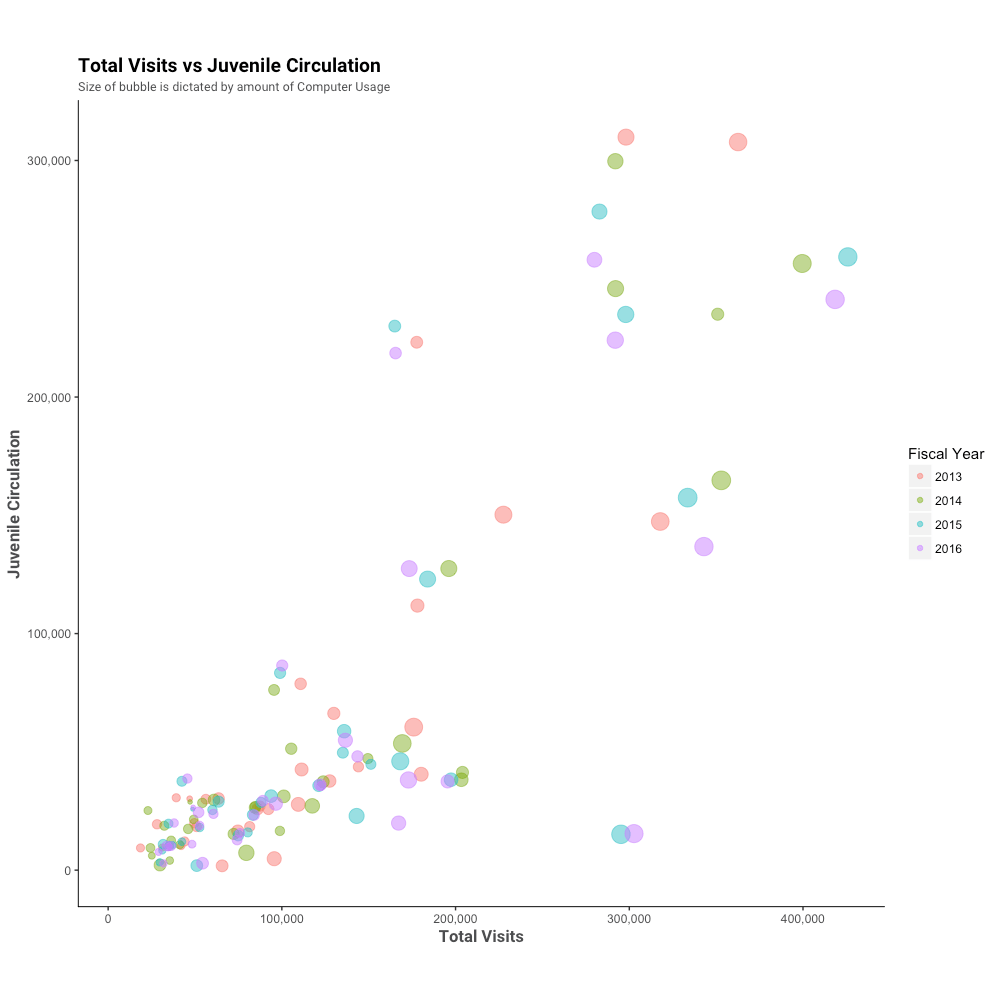Many more changes could be made to the graph based upon need including:

• Changing the colors
• Labeling extreme or interesting points
• Rotating labels

R is an extremely powerful tool when paired with packages like ggplot2. In addition, there are tools that can be used for more powerful visualizations. This can be pushed further by using packages such as R Shiny in order to create interactive applications.